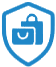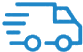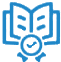Pythagoras Theorem – Oswaal Books and Learning Pvt Ltd# Pythagoras Theorem

Pythagoras Theorem (also known as Pythagorean Theorem) is a mathematical concept that describes how the sides of a right-angled triangle are related.

Pythagoras theorem tells that “The square of the hypotenuse side is equal to the sum of squares of the other two sides, in a right-angled triangle”. The sides of this triangle have been named Perpendicular, Base and Hypotenuse. The longest side of this triangle is hypotenuse to the opposite to 90o .

Pythagorean triples are another name for the sides of a right triangle. This theorem's formula and proof are presented with examples.

The Pythagoras theorem is a formula for calculating the length of an unknown side and the angle of a triangle. We can derive the base, perpendicular, and hypotenuse formulas using this theorem. Let's take a closer look at the Pythagorean theorem's mathematics.

History of Pythagoras' Theorem-Right Angle Triangle:

The theorem is named after Pythagoras, a Greek mathematician.

Formula for Pythagoras' Theorem

Consider the following triangle:

The perpendicular is denoted by "a."

The base is "b."

The hypotenuse is represented by the letter "c."

The Pythagoras Theorem formula, according to the definition, is as follows:

Perpendicular2 + Base2 = Hypotenuse2

a2 + b2 = c2

Because the side opposing the largest angle is the longest (known as Hypotenuse), the side opposite the right angle (90°) is the longest.

Theorem of Pythagoras

Consider three squares with sides a, b, and c that are attached to the three sides of a triangle with the same sides as indicated.

Theorem of Pythagoras –

The area of squares "a" and "b" equals the area of square "c."

Proof of Pythagoras' Theorem: A right-angled triangle ABC with a right angle at B.

To Demonstrate: AC2 = AB2 + BC2

Draw a perpendicular BD that intersects AC at D.

Proof of Pythagoras' Theorem

Proof: We all know what ADB and ABC stand for.

Therefore,

(Similar triangles' equivalent sides)

Alternatively, AB2 = AD + AC ……………………………...……. (1)

Moreover, BDC ABC

Therefore,

(Similar triangles' equivalent sides)

Alternatively, BC2 = CD AC ……………………………………. (2)

When we combine equations (1) and (2), we get

AB2 + BC2 = AD AB2 + BC2 = AD AB2 + BC2 = AD AB2 + BC2 = AD AB2 + BC2 =

AC (AD + CD) = AB2 + BC2

Because AD + CD Equals AC

As a result, AC2 = AB2 + BC2.

As a result, the Pythagorean theorem is established.

The Pythagorean theorem applies solely to right-angled triangles.

Pythagoras Theorem Applications

To determine whether or not the triangle is a right-angled triangle.

If the other two sides of a right-angled triangle are known, we can compute the length of any side.

To determine a square's diagonal.

Useful Pythagoras' theorem can be used to determine the sides of a right-angled triangle. We can find the third side of a right triangle if we know the two sides.

1. What is the best way to use it?

Remember the following formula to apply Pythagoras' theorem:

a2 + b2 = c2

1. How can you tell if a triangle is right-angled or not?

If we have the lengths of three sides of a triangle, we may apply the Pythagorean theorem to determine if the triangle is a right-angled triangle or not.

1. What is the Pythagorean Theorem formula?

In the case of a right-angled triangle, Pythagoras' formula is c2=a2+b2.

1. What is the hypotenuse formula?

The hypotenuse is the longest side of a right-angled triangle, opposite the right angle and perpendicular to the base. Let a, b, and c stand for the base, perpendicular, and hypotenuse, respectively. The hypotenuse formula will be c2 = (a2 + b2), as stated by Pythagoras.

Is the Pythagoras Theorem applicable to any triangle?

No, only the right-angled triangle is covered by this theorem.

1. What is Pythagoras' theorem good for?

The theorem can be used to calculate the steepness of mountains or hills. To calculate the distance between an observer and a location on the ground from a tower or building above which the observer is looking. It is mostly utilized in the building industry.Safe ShoppingQuick ShippingInclusive PricingTrusted Products